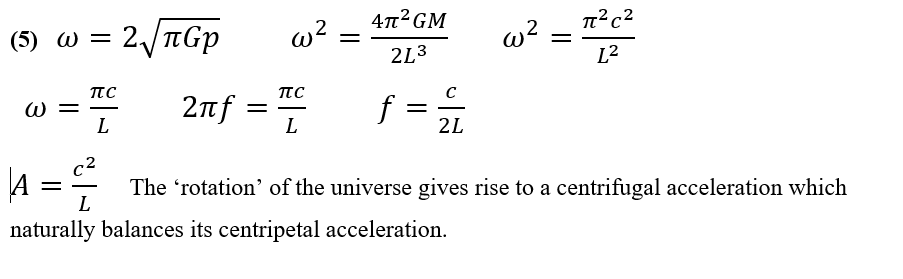Monday, 22 February 2021 17:05

## Equation 5Equation 5

Many items of rigorous mathematics with no obvious use can lie like buried swords awaiting discovery and application.

Kurt Gödel published another exact solution of the field equations of Einstein’s General Relativity in 1949.

‘Matter everywhere rotates relative to the compass of inertia with an angular velocity of twice the square root of pi times the gravitational constant times the density’.

This came too late to prevent Einstein’s capitulation to the idea of an expanding universe which became generally accepted from around 1930, but not necessarily by Gödel.

Gödel’s model of a non-expanding rotating universe with a metric of w = 2sqrt (pi G p) rapidly became ignored because no axis of rotation in the universe seemed observable, and, because it also predicted closed time-like curves.

However, as equation 5 shows, the Gödel metric has complete compatibility with the internal hypersphere metric of 2Gm/L = c^2. It supplies the centrifugal force apparent to rotating observers within a hypersphere.

The absence of an obvious axis of rotation in the universe arises because the gravitationally bound mega-structures such as galaxies and galactic clusters all rotate around the randomly orientated great circles of the Hopf Fibration that delineate the hypersphere giving it no overall angular momentum in any direction and hence no axis of rotation.

Closed time-like curves in a hyperspherical universe simply imply finite and unbounded time. The universe does not have to do the same things on every revolution, it does not imply eternal recurrence of exactly the same events, or travel back to the past.

Note that in substituting mass/volume for density p the equation uses hypersphere volume 2L^3/ pi, and that the frequency f of rotation comes out at c/2L, showing that for a hypersphere ‘rotation circumference’ equals twice the antipode length.

2Gm/L = c^2 represents the Interior Hypersphere Metric in the reference frame of a stationary interior observer.

w = 2sqrt (pi G p) represents the Interior Hypersphere Metric in the reference frame of a rotating interior observer.

Neither metric implies  real physical singularities or even  mere coordinate singularities..

Hypersphere Cosmology predicts that gravitationally bound independently moving megastructures within the universe, such as galactic clusters or isolated galaxies will have a rotation around the universe.

Each revolution of 360 degrees equals 360 x 3600 = 1.296 e6 arcseconds.
Each revolution takes 26 billion years 8.2 e17 seconds, at 3.154 e9 seconds per century this yields 2.6 e8 centuries.
Thus, galactic clusters move at 1.296 e6 / 2.6 e8 = 0.005 arcseconds per century
HC predicts the clusters will rotate around randomly orientated planes thus the maximum observable differences in angle between any pair equals ± 0.01 arcsecond per century.